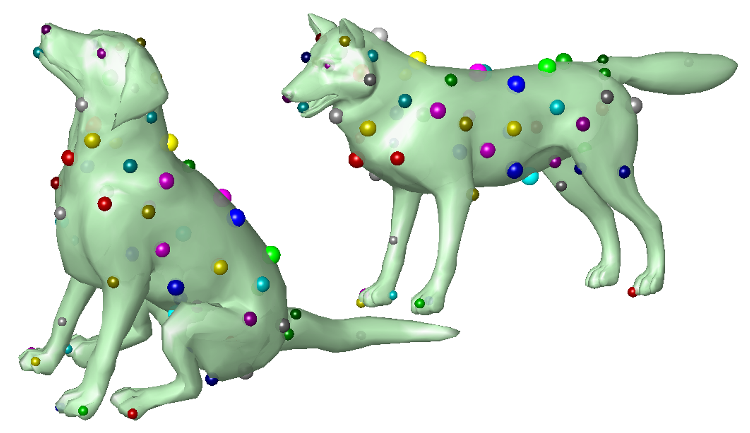PrincetonComputer SciencePIXL GroupPublications → [Lipman et al. 2009]
Möbius Voting for Surface Correspondence

ACM Transactions on Graphics (Proc. SIGGRAPH), August 2009

Yaron Lipman, Thomas FunkhouserCorrespondences found automatically between a dog and a wolf using Mobius Voting.
Abstract

The goal of our work is to develop an efficient, automatic algorithm for discovering point correspondences between surfaces th at are approximately and/or partially isometric.

Our approach is based on three observations. First, isometries are a subset of the Mobius group, which has low-dimensionality -- six degrees of freedom for topological spheres, and three for topological discs. Second, computing the Mobius transformat ion that interpolates any three points can be computed in closed-form after a zero-error mid-edge flattening to the complex pl ane. Third, deviations from isometry can be modeled by a transportation distance between corresponding points in that plane.

Motivated by these observations, we have developed a Mobius Voting algorithm that iteratively: 1) samples a triplet of three r andom points from each of two point sets, 2) uses the Mobius transformations defined by those triplets to map both point sets into a canonical coordinate frame on the complex plane, and 3) produces ``votes'' for predicted correspondences between the mu tually closest points with magnitude representing their estimated deviation from isometry. The result of this process is a fuz zy correspondence matrix, which is converted to a permutation matrix with simple matrix operations and output as a discrete se t of point correspondences with confidence values.

The main advantage of this algorithm is that it can find intrinsic point correspondences in cases of extreme deformation. Dur ing experiments with a variety of data sets, we find that it is able to find dozens of point correspondences between different object types in different poses fully automatically.

Paper
Citation

Yaron Lipman and Thomas Funkhouser.
"Möbius Voting for Surface Correspondence."
ACM Transactions on Graphics (Proc. SIGGRAPH) 28(3), August 2009.

BibTeX

```@article{Lipman:2009:MVF,
author = "Yaron Lipman and Thomas Funkhouser",
title = "M{\"o}bius Voting for Surface Correspondence",
journal = "ACM Transactions on Graphics (Proc. SIGGRAPH)",
year = "2009",
month = aug,
volume = "28",
number = "3"
}
```